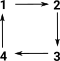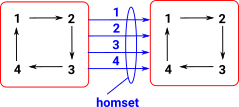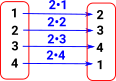# Maths - Cayley's Theorem

Here we want to relate permutations to other definitions of groups.

 So here we have a simple group, for example, in this case a cyclic group shown as a Cayley diagram:A common method when we want to understand a structure is to look at functions between the structures. In particular 'functors' which are functions which preserve structure.Here we apply one element of the group, in this case '2', known as a representable. This must 'play well' with the internal structure of the group.If we repeat this for all permutations we get a set of permutations (homset).## Other Representations

The group was represented by a Cayley diagram, in the example above, just to illustrate the situation graphically. Here we do the same example in terms of the group 'multiplication' operation.

 So lets take '2' as a representable then we can see that left 'multiplying' everything by 2 permutes the elements:Again if we repeat this for all permutations we get a set of permutations (homset).

## Further Information

Cayley's Theorem is a special case of the Yonada Lemma (see this page). This extends this idea to other structures. So any structure can be represented as a functor into set provided it is representable.Symmetry and the Monster - This is a popular science type book which traces the history leading up to the discovery of the largest symmetry groups.